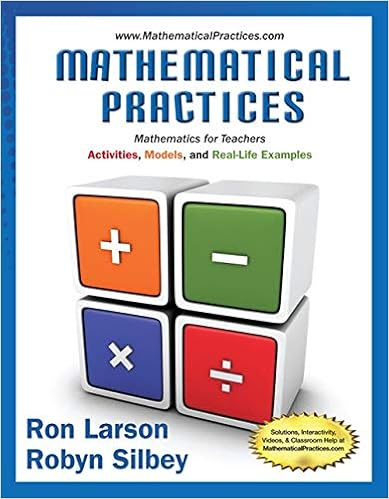# 4.01 Writing Assignment - Geometry Writing Assignment...

• Homework Help
• M.E.L57
• 3
• 100% (26) 26 out of 26 people found this document helpful

This preview shows page 1 out of 3 pages.

##### We have textbook solutions for you!
The document you are viewing contains questions related to this textbook.The document you are viewing contains questions related to this textbook.
Chapter 10 / Exercise 31
Mathematical Practices, Mathematics for Teachers: Activities, Models, and Real-Life Examples
LarsonExpert VerifiedUnformatted text preview: Geometry Writing Assignment – Introduction to Triangles Each problem is worth 5 points Total Points: 50 Solve the problems pertaining triangles. Be sure to show all work when needed. 1. Explain your reasoning. 2 RW = RU . Kendra disagrees. Is either of them correct? 3 RU is bigger than RW so Jordan must be wrong which means Kendra is correct in disagreeing with her. However, I do not believe I can say that 2/3 RU = RW because this is only true in certain cases (equilateral triangle) and we are not necessary told that this is the case. a. Based on the figure, Jordan says that b. If QW = 27 inches, find QT. 27 is 2/3 of the Total length 27/2 = 13.5 13.5 * 3 = 40.5 QT = 40.5 2. Jordan says that from the information supplied in the diagram, she can conclude that R is on the perpendicular bisector of QS . Robert disagrees. Is either of them correct? Explain your reasoning. Jordan is correct. We can see in the diagram that it bisects because of the two lines indicating the same length between the two new segments. 3. Franklin measured and labeled the angles of a triangle as shown. Jennifer says that at least one of his measures is incorrect. Explain in at least two different ways how Jennifer knows that this is true. One way Jennifer knows that this is true is because she by default all triangles angles are measured up to 180 degrees but in this case, all the angles equal to 268 degrees. Visually, one of the angles that is marked as 134 degrees is clearly way smaller than 90 degrees, meaning that there are two obtuse angles in this triangle "97 degrees and 134 degrees." 4. Joey says that triangle XYZ is obtuse. Robin disagrees because there are more acute angles then there are obtuse angles therefore it must be acute. Is either one of them correct? Explain your reasoning. Joey is correct about triangle XYZ being obtuse because Angle Z is more then 90 degrees but less then 190 degrees. 5. Compare and contrast the perpendicular bisectors and angle bisectors of a triangle. How are they alike? How are they different? Angle bisectors cut an angle into two congruent angles, and perpendicular bisectors are two lines that meet to form right angles at the midpoint of a line. They are different because one cuts angles and another cuts line segments. They are alike because they both bisect something in a triangle. 6. Compare and contrast the perpendicular bisectors, medians, and altitudes of a triangle. How are they alike? How are they different? All terms are very similar. They all go to an opposite vertex. Passing through the midpoint is another similarity. Both median and altitude start from one of the three vertex. Their differences are that perpendicular bisector must be ninety degrees, or it'll be a median. Altitude is the only term out of the three that do not start from a vertex. It must start from the base. 7a. Classify triangle ABC by its sides if side AB = 6x, side AC = 4x + 6 and side BC = 8x + 3. The perimeter of triangle ABC is 63. Show all your work. 6x + 8x + 4x = 18x 63 - 54 = 9 (6+3) So the side lengths are AB = 18, AC = 18, BC = 27 18 multiplied by 3 = 54 x=3 Triangle ABC is an isosceles triangle b. Explain your reasoning. Triangle ABC is an isosceles triangle because sides AB and AC are both the same length and when a triangle has two sides with the same length (two equal sides), that makes it classify as isosceles. 8. Is there enough information given to find the value of X. Explain your reasoning. There is not enough information to find the value of x. In order to determine the value of x, there would need to be markings indicating whether the segments drawn from the ray are congruent to each other. Since there are no markings indicating congruence, no conclusion can be made. ...
View Full Document

•••